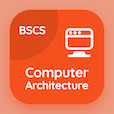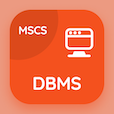Online CS Degree Courses

Computer Architecture MCQs

Computer Architecture MCQ - Topics

Learn Queuing Theory Multiple Choice Questions (MCQ Quiz), Queuing Theory quiz answers PDF to learn computer architecture course for computer architecture online classes. Computer Arithmetic Multiple Choice Questions and Answers (MCQs), Queuing Theory quiz questions for cheapest online computer science degree. Queuing Theory App Download: data dependences and hazards, queuing theory, major hurdle of pipelining, computer instructions representations test prep for online bachelor's degree computer science.

"For the operation of multiplication hardware needs minimum ALU of" MCQ PDF: queuing theory book with choices 16 bits, 32 bits, 64 bits, and 128 bits for cheapest online computer science degree. Study queuing theory quiz questions for merit scholarship test and certificate programs for computer majors.

## MCQs on Queuing Theory Quiz

MCQ: For the operation of multiplication hardware needs minimum ALU of

16 bits
32 bits
64 bits
128 bits

MCQ: The RAID devotes all of its resources for performing reconstruction, is a

online reconstruction
offline reconstruction
offline deconstruction
online deconstruction

MCQ: The primary challenge for every multiple-issue processors is trying to exploiting a large amount of

IP
FLP
FP
ILP

MCQ: The cost of the die can be calculated as

cost of die = cost of wafer\ dies per wafer + die yield
cost of die = cost of wafer\ dies per wafer - die yield
cost of die = cost of wafer\ dies per wafer * die yield
cost of die = cost of wafer\ dies per wafer \ die yield

MCQ: MIPS load word and store word instructions have the general form of

lw \$t1,offset_value(\$t2)
sw \$t1,offset_value(\$t2)
lw/sw \$t1,offset_value(\$t2)
ld/st \$t1,offset_value(\$t2)

### More Quizzes on Computer Architecture Book